# 06 - Unbalanced Datasets¶

version 1.3, June 2018

## Background¶

Unbalanced classification problems cause problems to many learning algorithms. These problems are characterized by the uneven proportion of cases that are available for each class of the problem.

Most classification algorithms will only perform optimally when the number of samples of each class is roughly the same. Highly skewed datasets, where the minority heavily outnumbered by one or more classes, haven proven to be a challenge while at the same time becoming more and more common.

One way of addresing this issue is by resampling the dataset as to offset this imbalance with the hope of arriving and a more robust and fair decision boundary than you would otherwise.

Resampling techniques are divided in two categories:

• Under-sampling the majority class(es)
• Over-sampling the minority class
In :
%matplotlib inline

import matplotlib as mpl
import matplotlib.pyplot as plt
import numpy as np
from sklearn.datasets import make_classification
from sklearn.decomposition import PCA
plt.style.use('ggplot')

In :
# Generate some data
X, y = make_classification(n_classes=2, class_sep=2, weights=[0.9, 0.1],
n_informative=3, n_redundant=1, flip_y=0,
n_features=20, n_clusters_per_class=1,
n_samples=1000, random_state=10)

# Instanciate a PCA object for the sake of easy visualisation
pca = PCA(n_components = 2)

# Fit and transform x to visualise inside a 2D feature space
x_vis = pca.fit_transform(X)

# Plot the original data

def plot_two_classes(X, y, subplot=False, size=(10, 10)):
# Plot the two classes
if subplot == False:
fig, subplot = plt.subplots(nrows=1, ncols=1, figsize=size)

subplot.scatter(X[y==0, 0], X[y==0, 1], label="Class #0",
alpha=0.5, s=70)
subplot.scatter(X[y==1, 0], X[y==1, 1], label="Class #1",
alpha=0.5, s=70)
subplot.legend()
return subplot

plot_two_classes(x_vis, y)

Out:
<matplotlib.axes._subplots.AxesSubplot at 0x7f5443d54da0>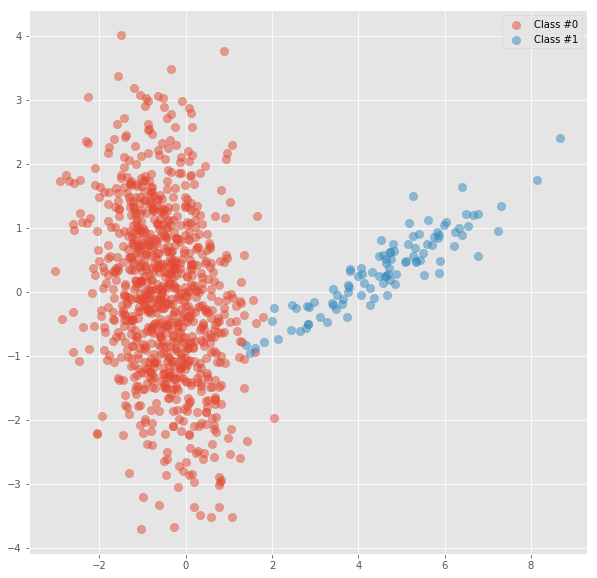In :
y.mean()

Out:
0.1

# Part 1: Under-sampling¶

## Random - Under-sampling¶

Randomly select a percentage of the negative class such that the resulting dataset is balanced

In :
n_samples = y.shape
print(n_samples)

1000

In :
n_samples_0 = (y == 0).sum()
n_samples_0

Out:
900
In :
n_samples_1 = (y == 1).sum()
n_samples_1

Out:
100
In :
n_samples_1 / n_samples

Out:
0.1

How many negatives cases should I select if I want a new dataset with 50% of positives?

0.5 = n_samples_1 / (n_samples_1 + n_samples_0_new)

(n_samples_1 + n_samples_0_new) = n_samples_1 / 0.5

In :
n_samples_0_new =  n_samples_1 / 0.5 - n_samples_1
n_samples_0_new

Out:
100.0
In :
n_samples_0_new_per = n_samples_0_new / n_samples_0
n_samples_0_new_per

Out:
0.1111111111111111

Create a filter to select n_samples_0_new_per from the negative class

In :
# Select all negatives
filter_ = y == 0

# Random sample
np.random.seed(42)
rand_1 = np.random.binomial(n=1, p=n_samples_0_new_per, size=n_samples)

# Combine
filter_ = filter_ & rand_1

In :
filter_.sum()

Out:
108

Also select all the positives

In :
filter_ = filter_ | (y == 1)

In :
filter_ = filter_.astype(bool)


plot

In :
plot_two_classes(x_vis[filter_], y[filter_])

Out:
<matplotlib.axes._subplots.AxesSubplot at 0x15efa139048>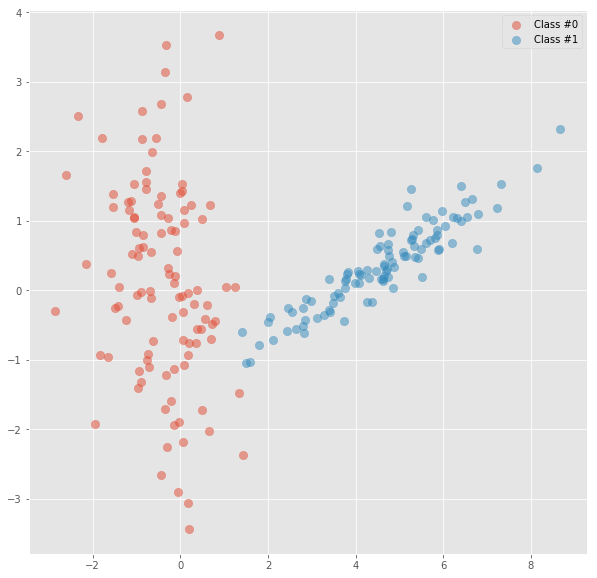Convert into a function

In :
def UnderSampling(X, y, target_percentage=0.5, seed=None):
# Assuming minority class is the positive
n_samples = y.shape
n_samples_0 = (y == 0).sum()
n_samples_1 = (y == 1).sum()

n_samples_0_new =  n_samples_1 / target_percentage - n_samples_1
n_samples_0_new_per = n_samples_0_new / n_samples_0

filter_ = y == 0

np.random.seed(seed)
rand_1 = np.random.binomial(n=1, p=n_samples_0_new_per, size=n_samples)

filter_ = filter_ & rand_1
filter_ = filter_ | (y == 1)
filter_ = filter_.astype(bool)

return X[filter_], y[filter_]

In :
for target_percentage in [0.1, 0.2, 0.3, 0.4, 0.5]:
X_u, y_u = UnderSampling(x_vis, y, target_percentage, 1)
print('Target percentage', target_percentage)
print('y.shape = ',y_u.shape, 'y.mean() = ', y_u.mean())
plot_two_classes(X_u, y_u, size=(5, 5))
plt.show()

Target percentage 0.1
y.shape =  1000 y.mean() =  0.1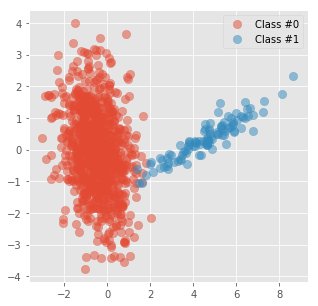Target percentage 0.2
y.shape =  510 y.mean() =  0.196078431373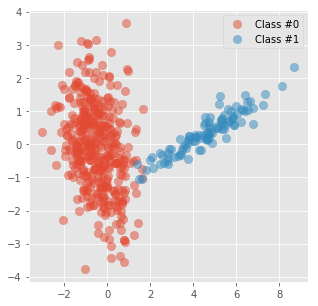Target percentage 0.3
y.shape =  343 y.mean() =  0.291545189504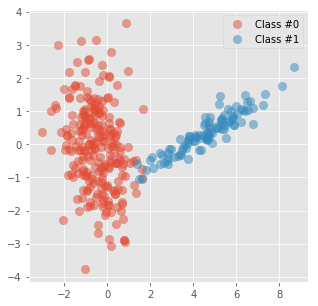Target percentage 0.4
y.shape =  249 y.mean() =  0.401606425703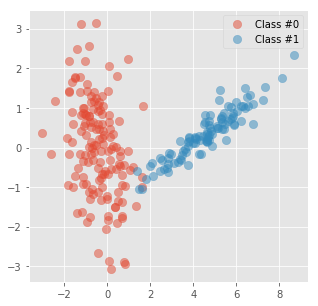Target percentage 0.5
y.shape =  199 y.mean() =  0.502512562814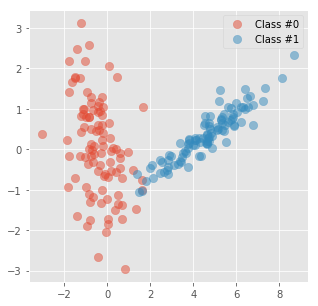### Comparing Under-Sampling with other models¶

• Fast to estimate
• Easy to understand

• Information of the negative class is lost
• Variance due to randomnes
• Need to define the target percentage

Identify and remove majority samples that form a Tomek link with minority samples.

Find the nearest neighbour of every point

In :
from sklearn.neighbors import NearestNeighbors

nn = NearestNeighbors(n_neighbors=2)
nn.fit(x_vis)
nns = nn.kneighbors(x_vis, return_distance=False)[:, 1]

In :
nns[0:10]

Out:
array([209, 633, 161, 610, 800, 268, 827, 606, 211, 940], dtype=int64)

Find if tomek. Use the target vector and the first neighbour of every sample point and looks for Tomek pairs. Returning a boolean vector with True for majority Tomek links.

In :
# Initialize the boolean result as false, and also a counter

# Loop through each sample of the majority class then we
# look at its first neighbour. If its closest neighbour also has the
# current sample as its closest neighbour, the two form a Tomek link.
for ind, ele in enumerate(y):

if ele == 1 | links[ind] == True:  # Keep all from the minority class
continue

if y[nns[ind]] == 1:

# If they form a tomek link, put a True marker on this
# sample, and increase counter by one.
if nns[nns[ind]] == ind:

In :
links.sum()

Out:
3
In :
filter_ = np.logical_not(links)

In :
print('y.shape = ',y[filter_].shape, 'y.mean() = ', y[filter_].mean())
plot_two_classes(x_vis[filter_], y[filter_])

y.shape =  997 y.mean() =  0.100300902708

Out:
<matplotlib.axes._subplots.AxesSubplot at 0x15efd2fc2b0>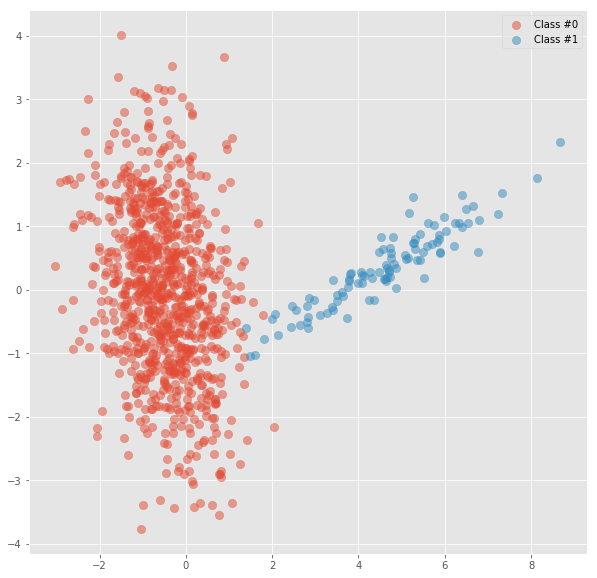• Creates a linearly-separable space

• Still a highly imbalance dataset

## Condensed Nearest Neighbour¶

The goal is to eliminate examples from the majority class that are much further away from the border

In :
# Import the K-NN classifier
from sklearn.neighbors import KNeighborsClassifier

In :
# Number of samples to extract in order to build the set S.
n_seeds_S = 51

# Size of the neighbourhood to consider to compute the
# average distance to the minority point samples.
size_ngh = 100

# Randomly get one sample from the majority class
np.random.seed(42)
maj_sample = np.random.choice(x_vis[y == 0].shape, n_seeds_S)
maj_sample = x_vis[y == 0][maj_sample]

In :
# Create the set C
# Select all positive and the randomly selected negatives
C_x = np.append(x_vis[y == 1], maj_sample, axis=0)
C_y = np.append(y[y == 1],  * n_seeds_S)

In :
# Create the set S
S_x = x_vis[y == 0]
S_y = y[y == 0]

In :
knn = KNeighborsClassifier(n_neighbors=size_ngh)

# Fit C into the knn
knn.fit(C_x, C_y)

# Classify on S
pred_S_y = knn.predict(S_x)

In :
# Find the misclassified S_y
idx_tmp = np.nonzero(y == 0)[np.nonzero(pred_S_y != S_y)]

In :
filter_ = np.nonzero(y == 1)
filter_ = np.concatenate((filter_, idx_tmp), axis=0)

In :
print('y.shape = ',y[filter_].shape, 'y.mean() = ', y[filter_].mean())
plot_two_classes(x_vis[filter_], y[filter_])

y.shape =  175 y.mean() =  0.571428571429

Out:
<matplotlib.axes._subplots.AxesSubplot at 0x15efd32b358>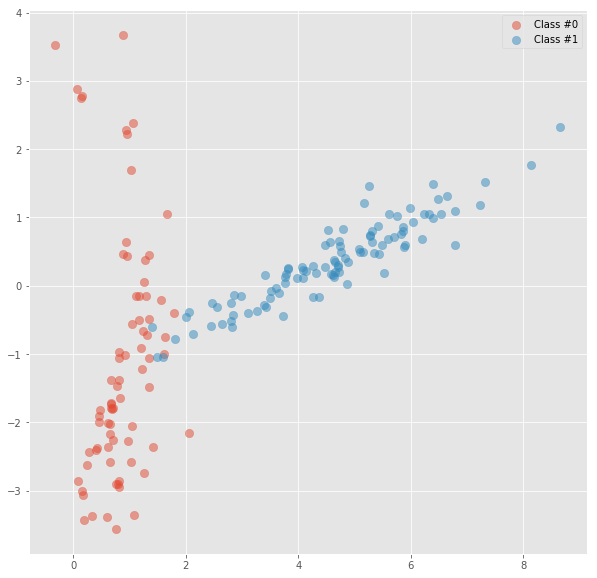In :
# Import the K-NN classifier
from sklearn.neighbors import KNeighborsClassifier
def CondensedNearestNeighbor(X, y, n_seeds_S=1, size_ngh=1, seed=None):
# Randomly get one sample from the majority class
np.random.seed(seed)
maj_sample = np.random.choice(X[y == 0].shape, n_seeds_S)
maj_sample = X[y == 0][maj_sample]
# Create the set C
# Select all positive and the randomly selected negatives
C_x = np.append(X[y == 1], maj_sample, axis=0)
C_y = np.append(y[y == 1],  * n_seeds_S)
# Create the set S
S_x = X[y == 0]
S_y = y[y == 0]
knn = KNeighborsClassifier(n_neighbors=size_ngh)

# Fit C into the knn
knn.fit(C_x, C_y)

# Classify on S
pred_S_y = knn.predict(S_x)
# Find the misclassified S_y
idx_tmp = np.nonzero(y == 0)[np.nonzero(pred_S_y != S_y)]

filter_ = np.nonzero(y == 1)
filter_ = np.concatenate((filter_, idx_tmp), axis=0)

return X[filter_], y[filter_]

In :
for n_seeds_S, size_ngh in [(1, 1), (100, 100), (50, 50), (100, 50), (50, 100)]:
X_u, y_u = CondensedNearestNeighbor(x_vis, y, n_seeds_S, size_ngh, 1)
print('n_seeds_S ', n_seeds_S, 'size_ngh ', size_ngh)
print('y.shape = ',y_u.shape, 'y.mean() = ', y_u.mean())
plot_two_classes(X_u, y_u, size=(5, 5))
plt.show()

n_seeds_S  1 size_ngh  1
y.shape =  386 y.mean() =  0.25906735751295334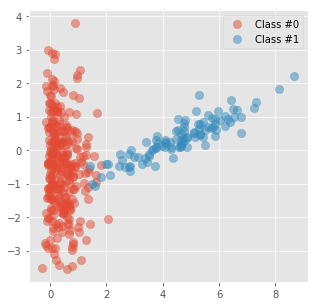n_seeds_S  100 size_ngh  100
y.shape =  100 y.mean() =  1.0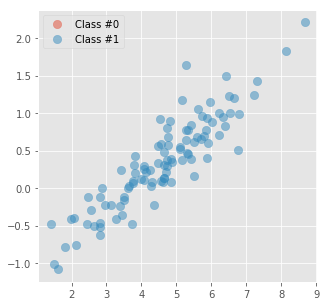n_seeds_S  50 size_ngh  50
y.shape =  112 y.mean() =  0.8928571428571429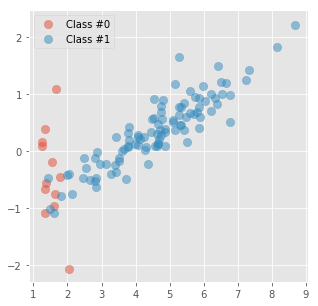n_seeds_S  100 size_ngh  50
y.shape =  102 y.mean() =  0.9803921568627451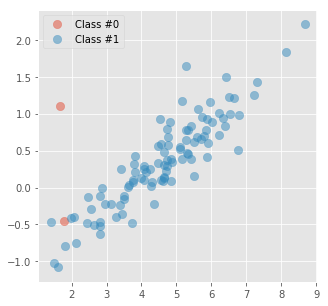n_seeds_S  50 size_ngh  100
y.shape =  246 y.mean() =  0.4065040650406504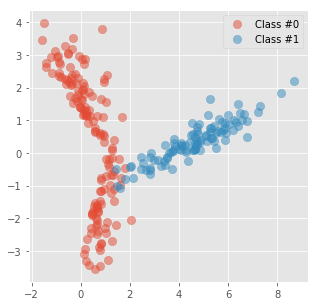### Comparing CondensedNearestNeighbor with other models¶

• Create Condensed space

• Variance due to randomnes
• Need to define the two parameters

## Other Under-sampling methods¶

• Under-sampling with Cluster Centroids
• NearMiss-(1 & 2 & 3)
• One-Sided Selection
• Neighboorhood Cleaning Rule

# Part 2: Over-Sampling¶

## Random over-sampling¶

Replicate the minority class examples to increase their relevance

In :
import random
def OverSampling(X, y, target_percentage=0.5, seed=None):
# Assuming minority class is the positive
n_samples = y.shape
n_samples_0 = (y == 0).sum()
n_samples_1 = (y == 1).sum()

n_samples_1_new =  -target_percentage * n_samples_0 / (target_percentage- 1)

np.random.seed(seed)
filter_ = np.random.choice(X[y == 1].shape, int(n_samples_1_new))
# filter_ is within the positives, change to be of all
filter_ = np.nonzero(y == 1)[filter_]

filter_ = np.concatenate((filter_, np.nonzero(y == 0)), axis=0)

return X[filter_], y[filter_]

In :
for target_percentage in [0.1, 0.2, 0.3, 0.4, 0.5]:
X_u, y_u = OverSampling(x_vis, y, target_percentage, 1)
print('Target percentage', target_percentage)
print('y.shape = ',y_u.shape, 'y.mean() = ', y_u.mean())
plot_two_classes(X_u, y_u, size=(5, 5))
plt.show()

Target percentage 0.1
y.shape =  1000 y.mean() =  0.1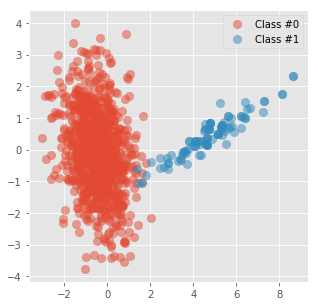Target percentage 0.2
y.shape =  1125 y.mean() =  0.2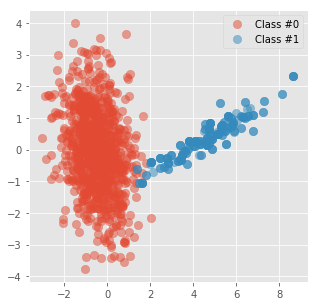Target percentage 0.3
y.shape =  1285 y.mean() =  0.299610894942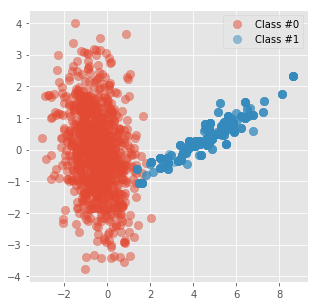Target percentage 0.4
y.shape =  1500 y.mean() =  0.4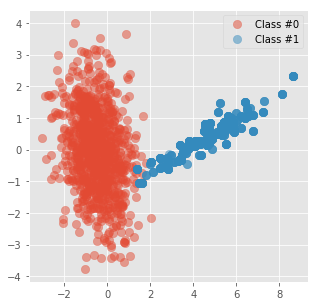Target percentage 0.5
y.shape =  1800 y.mean() =  0.5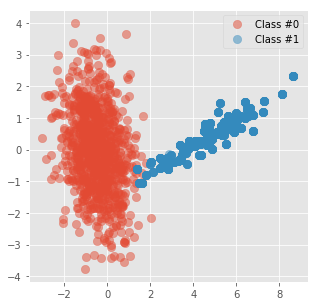### Comparing Over-Sampling with other models¶

• Fast to estimate
• Easy to understant
• No information of the majority class is lost

• No new information is added
• Hurts the generalization capacity
• Variance due to randomnes
• Need to define the target percentage

## SMOTE¶

SMOTE (Chawla et. al. 2002) is a well-known algorithm to fight this problem. The general idea of this method is to artificially generate new examples of the minority class using the nearest neighbors of these cases. Furthermore, the majority class examples are also under-sampled, leading to a more balanced dataset.

In :
# Number of nearest neighbours to used to construct synthetic samples.
k = 5

from sklearn.neighbors import NearestNeighbors
nearest_neighbour_ = NearestNeighbors(n_neighbors=k + 1)

In :
# Look for k-th nearest neighbours, excluding, of course, the
# point itself.#
nearest_neighbour_.fit(x_vis[y==1])

Out:
NearestNeighbors(algorithm='auto', leaf_size=30, metric='minkowski',
metric_params=None, n_jobs=1, n_neighbors=6, p=2, radius=1.0)
In :
# Matrix with k-th nearest neighbours indexes for each minority
# element.#
nns = nearest_neighbour_.kneighbors(x_vis[y==1],
return_distance=False)[:, 1:]


Example of the nns of two cases

In :
def base_smote_plot(sel, nns):
fig, subplot = plt.subplots(nrows=1, ncols=1, figsize=(10, 10))

for i in range(len(sel)):
# plot the select sample
subplot.scatter(x_vis[y==1, 0][sel[i]], x_vis[y==1, 1][sel[i]],
alpha=1., s=70)
# plot the neighbors
subplot.scatter(x_vis[y==1, 0][nns[sel[i]]],
x_vis[y==1, 1][nns[sel[i]]],
alpha=0.5, s=70)

# plot the lines
for nn in nns[sel[i]]:
plt.plot([x_vis[y==1, 0][sel[i]], x_vis[y==1, 0][nn]],
[x_vis[y==1, 1][sel[i]], x_vis[y==1, 1][nn]],
'r--')

xlim = subplot.get_xlim()
ylim = subplot.get_ylim()
subplot.scatter(x_vis[y==1, 0], x_vis[y==1, 1], alpha=0.1, s=70)
subplot.set_xlim(xlim)
subplot.set_ylim(ylim)
return subplot
base_smote_plot(,nns)

Out:
<matplotlib.axes._subplots.AxesSubplot at 0x15efd4092b0>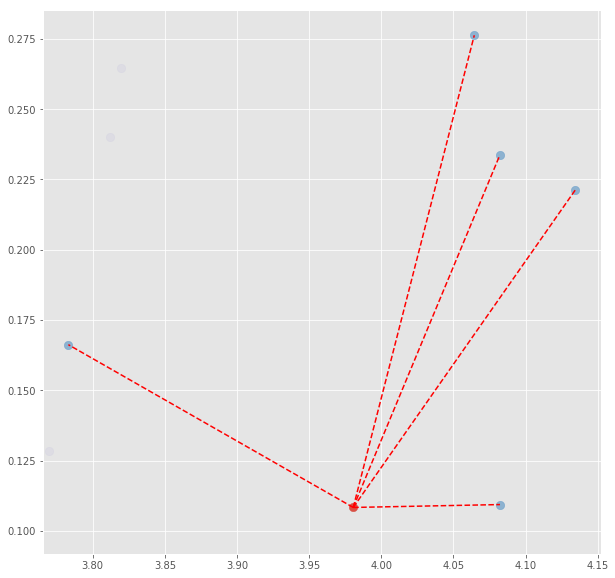In :
# Create syntetic sample for 12

# Select one random neighbor
np.random.seed(3)
nn_ = np.random.choice(nns)

x_vis[y==1][nn_]

Out:
array([ 4.06447682,  0.27624239])
In :
# Take a step of random size (0,1) in the direction of the
# n nearest neighbours
np.random.seed(5)
step = np.random.uniform()

# Construct synthetic sample
new = x_vis[y==1] - step * (x_vis[y==1] - x_vis[y==1][nn_])
new

Out:
array([ 3.99919087,  0.14561291])
In :
plot_ = base_smote_plot(,nns)
plot_.scatter(new, new, alpha=1., s=400, marker="*")

Out:
<matplotlib.collections.PathCollection at 0x15efd7cfa58>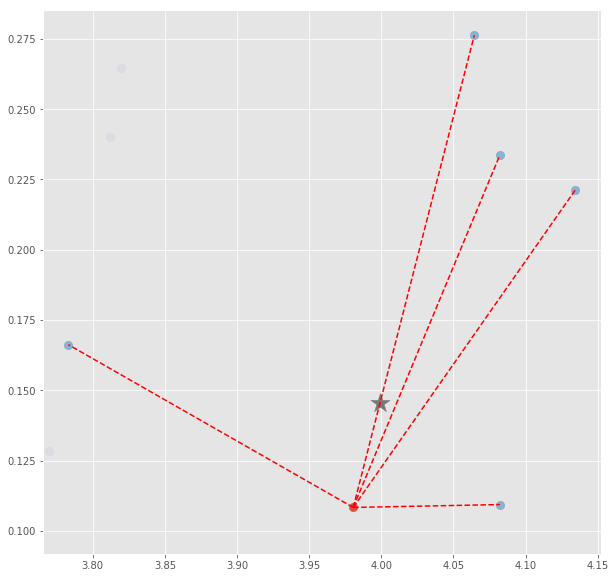In :
# Select one random neighbor
np.random.seed(5)
nn_2 = np.random.choice(nns)
np.random.seed(1)
step = np.random.uniform()
# Construct synthetic sample
new2 = x_vis[y==1] - step * (x_vis[y==1] - x_vis[y==1][nn_2])

plot_ = base_smote_plot(,nns)
plot_.scatter(new, new, alpha=1., s=400, marker="*")
plot_.scatter(new2, new2, alpha=1., s=400, marker="*")

Out:
<matplotlib.collections.PathCollection at 0x7fd7eed5d908>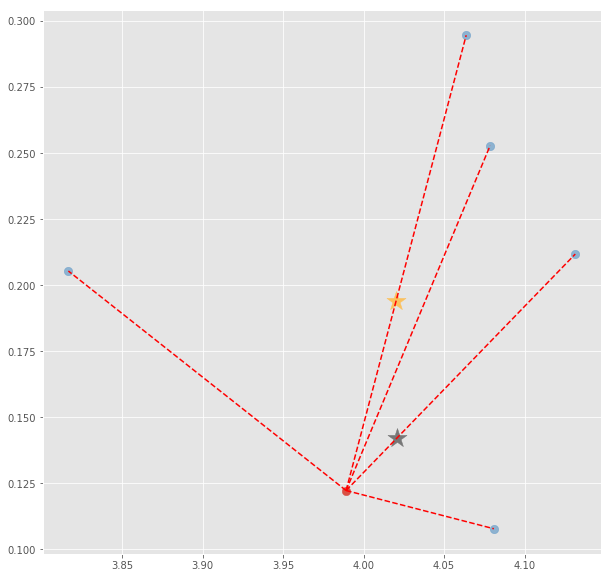Apply to all cases

Estimate number of synthetic cases to create

In :
target_percentage = 0.5
n_samples_1_new =  -target_percentage * n_samples_0 / (target_percentage- 1) - n_samples_1
n_samples_1_new = int(n_samples_1_new)
n_samples_1_new

Out:
800
In :
# A matrix to store the synthetic samples
new = np.zeros((n_samples_1_new, x_vis.shape))

In :
# Select examples to use as base
np.random.seed(34)
sel_ = np.random.choice(y[y==1].shape, n_samples_1_new)

In :
# Define random seeds (2 per example)
np.random.seed(64)
nn__ = np.random.choice(k, n_samples_1_new)
np.random.seed(65)
steps = np.random.uniform(size=n_samples_1_new)

In :
# For each selected examples create one synthetic case
for i, sel in enumerate(sel_):
# Select neighbor
nn_ = nn__[i]
step = steps[i]
new[i, :] = x_vis[y==1][sel] - step * (x_vis[y==1][sel] - x_vis[y==1][nn_])

In :
new

Out:
array([[4.99956657, 0.45629856],
[4.9262738 , 0.41983964],
[4.64508466, 0.25702488],
...,
[5.58945487, 0.51102965],
[5.47529954, 0.39266754],
[5.09802765, 0.36978454]])
In :
plot_ = plot_two_classes(x_vis, y, size=(10, 10))
plot_.scatter(new[:, 0], new[:, 1], alpha=0.5, s=150, marker='*')

Out:
<matplotlib.collections.PathCollection at 0x7fd7ece825c0>